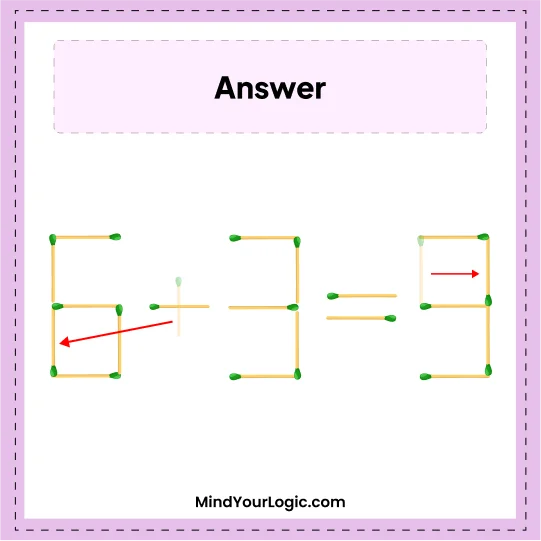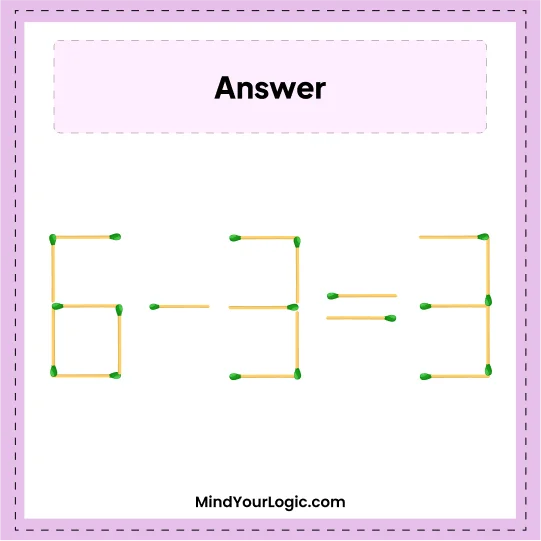# Move 2 Matchstick to get 5+3=5 - Matchstick Puzzle

###### 34.Matchstick Puzzles
`Challenge yourself with this matchstick puzzle. Move 2 matchsticks to get correct equation.`•

Explanation :

` The one stick of + sign will move to 5 which becomes 6 and one stick of 5 shifts back, it becomes 3. So, the correct equation we get is 6 - 3 = 3.`